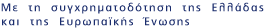Now showing items 1-3 of 3

• #### Identification of classes of H-matrices ﻿

(2010)
H-matrices play an important role in the theory and applications. Different algorithms to determine H-matrices can be found in the literature. In  an algorithm is given, and this algorithm can determine if an irreducible ...
• #### Is A is an element of C-n,C-n a general H-matrix? ﻿

(2012)
H-matrices play an important role in the theory and applications of Numerical Linear Algebra. So, it is very useful to know whether a given matrix A is an element of C-n,C-n, usually the coefficient of a complex linear ...
• #### The matrix analogue of the scalar AOR iterative method ﻿

(2015)
The Accelerated Overrelaxation (AOR) and the Generalized AOR (GAOR) iterative methods for the solution of linear systems of algebraic equations (Ax = b, A is an element of C-nxn, det(A) not equal 0, b is an element of C-n) ...